TUTORIALS
TUTORIALS HOME

GENERAL MATH
NUMBER SETS
ABSOLUTE VALUE & INEQUALITIES
SETS & INTERVALS
FRACTIONS
POLYNOMIALS
LINEAR EQUATIONS
GEOMETRY
FINITE SERIES
TRIGONOMETRY
EXPONENTS
LOGARITHMS
INDUCTION

CALCULUS
LIMITS
DERIVATIVES
RELATED RATES & OPTIMIZATION
CURVE SKETCHING
INTEGRALS
AREA & VOLUME
INVERSE FUNCTIONS

MAIN
HOME
TESTS
TUTORIALS
SAMPLE PROBLEMS
COMMON MISTAKES
STUDY TIPS
GLOSSARY
CALCULUS APPLICATIONS
MATH HUMOUR

### AREA & VOLUME TUTORIAL

This tutorial exhibits some of the applications of integral calculus. Although these are only some of the applications of integration, they will give you an idea of how calculus can be used to solve problems in other areas of mathematics.

# Areas Between Curves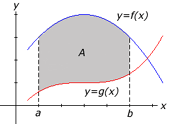The area A of the region bounded by the curves, f(x) and g(x), and the lines x=a and x=b, where f and g are continuous and f(x)>g(x) for all x in the interval [a, b], is given by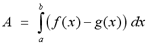The figure to the right illustrates the region that is given by the definition above. To make the image clearer, the curve f(x) is shown in blue and the curve g(x) is shown in red.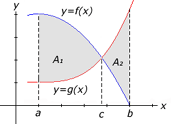In some problems, the curves may intersect so that f(x) is not greater than g(x) over the entire interval [a, b]. The graph to the right illustrates this situation. In this case, we must find the point of intersection, c, between the two curves. To find the point of intersection c, we set f(x) = g(x) and solve the resulting equation for x. To find the area, we split the region A between the curves into 2 separate regions, A1, bounded by f(x) and g(x) and the lines x=a and x=c, and A2, bounded by g(x) and f(x) and the lines x=c and x=b. To find the area of A, we calculate the area of each individual region and take the sum of the results.

For the curve to the right, the equation for the area of the region would be given by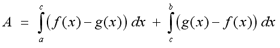Note: This equation depends on the nature of the question. Not all problems will use this same equation to determine the area. For example, if the curve g(x) is greater than f(x) on [a,c), and less than f(x) on (c,b], we would switch the f(x) with g(x) in the equation above.

In other problems, the region may be bounded by the curves f(x) and g(x) over part of the interval [a,b] but may be bounded by only a single curve for another part of the interval. The figure below illustrates this situation.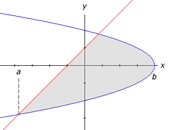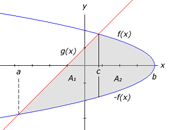In a problem like this, there are two possible solutions.

a) The first method is to find the point of intersection, c, between the two curves. We split the region A between the curves into 2 separate regions, A1, bounded by f(x) and g(x) and the lines x=a and x=c, and A2, bounded by +f(x) and -f(x) and the lines x=c and x=b. The image to the right illustrates the first method of solving this type of area problem. The formula to the below shows how the area would be calculated for this specific example.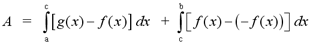Note: Like the problem above, this equation depends on the nature of the question. You must recognize which function defines the upper boundary and which defines the lowers boundary over a certain interval.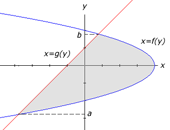b) The second method involves rewriting the problem so that it is in terms of y rather than x. We express each curve in terms of y, so that we have f(y) for the right boundary and g(y) for the left boundary. We must find the y coordinates of the intersection points of the curves. We let y=b represent the higher intersection point and y=a represent the lower intersection point. The image to the right illustrates the second approach to solving the example above. The equation below shows how the area would be calculated for this specific example.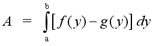Note: Once again, this equation depends on the nature of the question.

In fact, this method can be used to solve any area problems. It may often be simpler to solve the integrals when the functions in terms of y rather than x. If the problem seems too difficult, we can always try to solve it in terms of y.

# Examples

1 | Find the area of the region between the curves
2 | Find the area of the region between the intersecting curves
3 | Find the area of the region between the curves, with respect to y

# Volumes

Integration also allows us to calculate the volumes of solids. Let S be a solid that lies between x=a and x=b. Let the continuous function A(x) represent the cross-sectional area of S in the plane through the point x and perpendicular to the x-axis. The volume of S is given by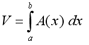Now that we have the definition of volume, the challenging part is to find the function of the area of a given cross section. This process is quite similar to finding the area between curves.

Most volume problems that we will encounter will be require us to calculate the volume of a solid of rotation. These are solids that are obtained when a region is rotated about some line. A typical volume problem would ask, "Find the volume of the solid obtained by rotating the region bounded by the curve(s) about some specified line." Since the region is rotated about a specific line, the solid obtained by this rotation will have a disk-shaped cross-section. We know from simple geometry that the area of a circle is given by A = p r2. For each cross-sectional disk, the radius is determined by the curves that bound the region. If we sketch the region bounded by the given curves, we can easily find a function to determine the radius of the cross-sectional disk at point x.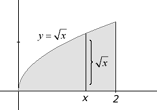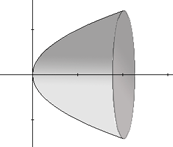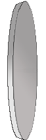The figures above illustrates this concept. The figure to the left shows the region bounded by the curve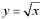and the x-axis and the lines x = 0 and x = 2. The figure in the center shows the 3-dimensional solid that is formed when the region from the first figure is rotated about the x-axis. The figute to the right shows a typical cross-sectional disk. A disk for a given value x between 0 and 2 will have a radius of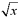. The area of the disk is given by A(x) = p ()2 or equivalently, A(x) = px. Once we find the area function, we simply integrate from a to b to find the volume. The examples below will show complete solutions to finding the area of a given solid.

# Variations of Volume Problems

There are several variations in these types of problems. The first factor that can vary in a volume problem is the axis of rotation. What if the region from the figure above was rotated about the y-axis rather than the x-axis? We would end up with a different function for the radius of a cross-sectional disk. The function would be written with respect to y rather than x, so we would have to integrate with respect to y. In general, we can use the following rule.

If the region bounded by the curves f(x) and g(x) and the lines x=a and x=b is rotated about an axis parallel to the x-axis, write the integral with respect to x. If the axis of rotation is parallel to the y-axis, write the integral with respect to y.

The second factor that can vary in volume problems is the radius of a typical cross-sectional disk. Suppose that the region is bounded by two curves, f(x) and g(x), that both vary between x=a and x=b. The solid that is created by rotating this region about some specified line will have a hole in the center. The radius of a cross-sectional disk will be determined by two functions, rather than a single function. This variation creates two separate styles of problems.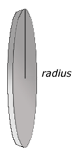The Disk Method : The disk method is used when the cross sections are disk shaped. The radius of a cross section is determined by a single function, f(x). The area of the disk is given by the formula, A(x) = p (radius)2. The figure to the right shows a typical cross-sectional disk.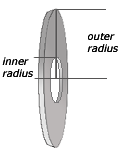The Washer Method : The washer method is used when the cross sections are washer shaped. The radius of a cross section is determined by a two functions, f(x) and g(x). This gives us two separate radii, an outer radius, from f(x) to the axis of rotation and an inner radius from g(x) to the axis of rotation. The area of the washer is given by the formula, A(x) = p [ (outer radius)2-(inner radius)2 ]. The figure to the left shows a typical cross-sectional washer.

# Examples

4 | Find the volume of the solid (axis of rotation parallel to y-axis)
5 | Find the volume of the solid using the washer method

# Volumes By Cylindrical Shells

There is another method to solve volume problems, in addition to the methods described in the section above. The method of cylindrical shells is sometimes simpler to use than the previous methods. For example, suppose we want to find the volume of the solid obtained by rotating about the y-axis, the region bounded by the y=3x-x3 and the line y=0. Since the axis of rotation is parallel to the y-axis, we must integrate the area function with respect to y. To do this, We must solve the cubic function for x in terms of y. This would be rather difficult. However, we will soon see that this problem can be solved quite easily using the method of cylindrical shells.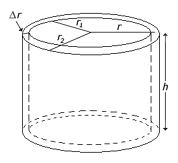The image to the right shows a cylindrical shell with outer radius r2, inner radius r1 and height h. We can calculate the volume of the shell by finding the volume of the inner cylinder, V1 and subtracting it from the volume of the outer cylinder, V2. Recall from the geometry tutorial, that the volume of a cylinder is given by the formula V = pi r2h.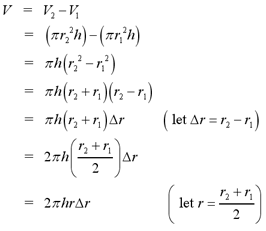We let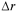represent the thickness of the cylindrical shell and r represent the average radius of the shell. In summary, the volume of a cylindrical shell is given by the following formula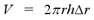Now that we have covered the concept of a cylindrical shell, we can apply it to general volume problems. The idea behind the method of cylindrical shells is to think of a 3-dimensional solid as a collection of cylindrical shells. To find the volume of the solid, we must integrate the formula for the volume of a cylindrical shell. However, the formula for the volume of the cylindrical shell will vary with each problem. We must find functions for the height and the radius of the cylindrical shell at x. Suppose the functions are h(x) for height and r(x) for radius. The volume of the solid obtained by rotating a region about a specific line from a to b is given by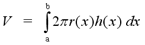This may seem complicated, but after a few examples the method will be much clearer.

# Examples

6 | Find the volume of the solid using the method of cylindrical shells
7 | Find the volume of the solid

For more practice with the concepts covered in this tutorial, visit the Area and Volume Problems page at the link below. The solutions to the problems will be posted after these chapters are covered in your calculus course.

To test your knowledge of applications of integration problems, try taking the general area and volume test on the iLrn website or the advanced area and volume test at the link below.

Area & Volume Problems
General Area & Volume Test on iLrn
Advanced Area & Volume Test

|Top of Page |

COURSE HOMEPAGES
MATH 1036
MATH 1037

FACULTY HOMEPAGES
Alex Karassev
Ted Chase